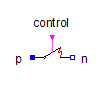Frequency Sweep Voltage Source - MapleSim Help

Frequency Sweep Voltage Source

Voltage source with integrated frequency sweepDescription The Frequency Sweep Voltage Source component provides a polyphase constant RMS phase voltage $V$ and phase angle $\mathrm{\phi }$, whereas the frequency sweeps from ${f}_{\mathrm{start}}$ to ${f}_{\mathrm{stop}}$ with $\mathrm{duration}$. The frequency sweeps such way that on a logarithmic frequency scale, the frequency curve appears linear.Equations $i=\mathrm{plug_p.pin.i}$ $\mathrm{\omega }=\mathrm{Diff}\left(\mathrm{plug_p.reference.gamma},t\right)$ $v=\mathrm{plug_p.pin.v}-\mathrm{plug_n.pin.v}$Variables

 Name Value Units Description Modelica ID $v$ Complex voltage v ${\mathrm{abs}}_{v}$  $V$ Magnitude of complex voltage abs_v ${\mathrm{arg}}_{v}$  $\mathrm{rad}$ Argument of complex voltage arg_v $i$ Complex current i ${\mathrm{abs}}_{i}$  $A$ Magnitude of complex current abs_i ${\mathrm{arg}}_{i}$  $\mathrm{rad}$ Argument of complex current arg_i $P$ {Modelica.ComplexMath.real(v[k]  Modelica.ComplexMath.conj(i[k])) for k in 1:m} $W$ Active power P ${P}_{\mathrm{total}}$ $\mathrm{sum}\left(P\right)$ $W$ Total active power P_total $Q$ {Modelica.ComplexMath.imag(v[k]  Modelica.ComplexMath.conj(i[k])) for k in 1:m} $\mathrm{var}$ Reactive power Q ${Q}_{\mathrm{total}}$ $\mathrm{sum}\left(Q\right)$ $\mathrm{var}$ Total reactive power Q_total $S$ {Modelica.ComplexMath.'abs'(v[k]  Modelica.ComplexMath.conj(i[k])) for k in 1:m} $VA$ Magnitude of complex apparent power S ${S}_{\mathrm{total}}$  $VA$ Magnitude of total complex apparent power S_total $\mathrm{pf}$ {cos(Modelica.ComplexMath.arg(Complex(P[k], Q[k]))) for k in 1:m} Power factor pf $\mathrm{\omega }$ $\frac{\mathrm{rad}}{s}$ Angular velocity of reference frame omega ${\mathrm{plugToPins}}_{p}$ plugToPins_p ${\mathrm{plugToPins}}_{n}$ plugToPins_n $\mathrm{\gamma }$ ${\mathrm{plug}}_{p}\cdot \mathrm{reference}\cdot \mathrm{\gamma }$ $\mathrm{rad}$ gamma $f$ $\mathrm{voltageSource}\cdot f$ $\mathrm{Hz}$ Actual frequency f $\mathrm{voltageSource}$ voltageSource $\mathrm{logFrequencySweep}$ logFrequencySweep $\mathrm{const}$ const

 $\mathrm{Modelica}\cdot \mathrm{ComplexMath}\cdot \prime \mathrm{abs}\prime \left(v\right)$

 $\mathrm{Modelica}\cdot \mathrm{ComplexMath}\cdot \mathrm{arg}\left(v\right)$

 $\mathrm{Modelica}\cdot \mathrm{ComplexMath}\cdot \prime \mathrm{abs}\prime \left(i\right)$

 $\mathrm{Modelica}\cdot \mathrm{ComplexMath}\cdot \mathrm{arg}\left(i\right)$

 $\sqrt{{P}_{\mathrm{total}}^{2}+{Q}_{\mathrm{total}}^{2}}$Connections

 Name Description Modelica ID ${\mathrm{plug}}_{p}$ Positive quasi-static polyphase plug plug_p ${\mathrm{plug}}_{n}$ Negative quasi-static polyphase plug plug_nParameters

 Name Default Units Description Modelica ID $m$ $3$ Number of phases m ${f}_{\mathrm{start}}$ $\mathrm{Hz}$ Start sweep frequency fStart ${f}_{\mathrm{stop}}$ $\mathrm{Hz}$ Stop sweep frequency fStop ${T}_{0}$ $0$ $s$ Start time of frequency sweep startTime duration $s$ Duration of frequency sweep duration ${V}_{\mathrm{rms}}$ $V$ RMS voltage of the source V $\mathrm{\phi }$  $\mathrm{rad}$ Phase shift of the source phi

 $-\mathrm{Modelica}\cdot \mathrm{Electrical}\cdot \mathrm{MultiPhase}\cdot \mathrm{Functions}\cdot \mathrm{symmetricOrientation}\left(m\right)$Modelica Standard Library The component described in this topic is from the Modelica Standard Library. To view the original documentation, which includes author and copyright information, click here.